Origin Of Quantum Mechanics MCQ Level - 2

# Origin Of Quantum Mechanics MCQ Level - 2

Test Description

## 10 Questions MCQ Test Topic wise Tests for IIT JAM Physics | Origin Of Quantum Mechanics MCQ Level - 2

Origin Of Quantum Mechanics MCQ Level - 2 for IIT JAM 2023 is part of Topic wise Tests for IIT JAM Physics preparation. The Origin Of Quantum Mechanics MCQ Level - 2 questions and answers have been prepared according to the IIT JAM exam syllabus.The Origin Of Quantum Mechanics MCQ Level - 2 MCQs are made for IIT JAM 2023 Exam. Find important definitions, questions, notes, meanings, examples, exercises, MCQs and online tests for Origin Of Quantum Mechanics MCQ Level - 2 below.
Solutions of Origin Of Quantum Mechanics MCQ Level - 2 questions in English are available as part of our Topic wise Tests for IIT JAM Physics for IIT JAM & Origin Of Quantum Mechanics MCQ Level - 2 solutions in Hindi for Topic wise Tests for IIT JAM Physics course. Download more important topics, notes, lectures and mock test series for IIT JAM Exam by signing up for free. Attempt Origin Of Quantum Mechanics MCQ Level - 2 | 10 questions in 45 minutes | Mock test for IIT JAM preparation | Free important questions MCQ to study Topic wise Tests for IIT JAM Physics for IIT JAM Exam | Download free PDF with solutions
 1 Crore+ students have signed up on EduRev. Have you?
Origin Of Quantum Mechanics MCQ Level - 2 - Question 1

### An atom has n = 1 and n = 2 levels filled. How many electrons does the atom have?

Detailed Solution for Origin Of Quantum Mechanics MCQ Level - 2 - Question 1

Number of electrons in nth orbit is given by 2n2
∴  total electrons is = 2(1)2 + 2(22)
= 2 + 8 = 10

Origin Of Quantum Mechanics MCQ Level - 2 - Question 2

### In a hydrogen atom, the ratio of the wavelengths of lyman-α radiation (in n = 2 to n =1) to Balmer-α radiation (n = 3 → n = 2) is

Detailed Solution for Origin Of Quantum Mechanics MCQ Level - 2 - Question 2

Transition to n=1 and n=2 are refered to as Lyman and Balmer series.

For Lyman Series wavelength will be longest when energy the electron has transition from n=2 to n=1 level.
For Balmer  Series wavelength will be longest when  energy the electron has transition from n=3 to n=2 level.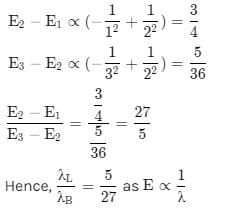Origin Of Quantum Mechanics MCQ Level - 2 - Question 3

### de-Broglie hypothesized that the momentum and wavelength of a free massive particle are related by

Detailed Solution for Origin Of Quantum Mechanics MCQ Level - 2 - Question 3

de-Broglie wave length, λ = h/p, where h is the Planck's constant.

The correct answer is: Planck’s constant

Origin Of Quantum Mechanics MCQ Level - 2 - Question 4

Which of the following is true? When metallic surface is irradiated with white light

Detailed Solution for Origin Of Quantum Mechanics MCQ Level - 2 - Question 4

Explanation : White light does not produce any photoelectric effect because the range of wavelength in white light is (400 – 700)nm. The energy corresponding to this range of wavelength is small in comparison to the work function of any metal in general.

The correct answer is: No photo-current observed.

Origin Of Quantum Mechanics MCQ Level - 2 - Question 5

Positronium is an atom formed by an electron and a positron (anti electron), it is similar to the hydrogen atom with proton being replaced by a positron. If this positronium atom makes a transition from n = 3 to n = 1 the corresponding photon energy would be :

Detailed Solution for Origin Of Quantum Mechanics MCQ Level - 2 - Question 5

The reduced mass of positronium is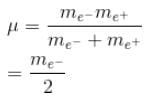In the case of hydrogen atom, for simplification we take the reduced mass of the electron and proton system to be me.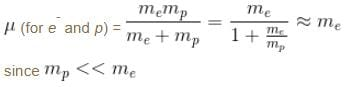So, the only difference between the hydrogen and positronium atom is that the positronium atom has the energy levels half of the hydrogen atom.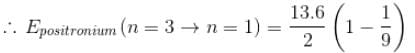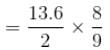= 6.0 eV
The correct answer is: 6.0 eV

Origin Of Quantum Mechanics MCQ Level - 2 - Question 6

The energy required to remove both electrons from the helium atom in its ground state is 79.0 eV. How much energy is required to ionize helium? (i.e. to remove single electron)

Detailed Solution for Origin Of Quantum Mechanics MCQ Level - 2 - Question 6

Consider He+ which is a hydrogen like atom. We can apply the Bohr theory to see that energy required to go from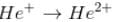What we need is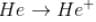Given is the total energy required to get He2+ from He.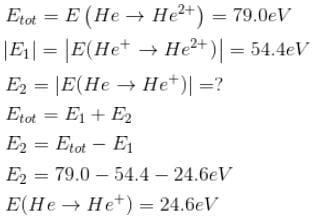We cannot apply Bohr theory directly to Helium because it is not a hydrogen like atom.

The correct answer is: 24.6 eV

Origin Of Quantum Mechanics MCQ Level - 2 - Question 7

A particle with initial K.E = E and de-Broglie wavelength λ enters a region in which it has potential energy V. What is the particle’s new de-Broglie wavelength?

Detailed Solution for Origin Of Quantum Mechanics MCQ Level - 2 - Question 7

In the initial case (free particle)
V = 0
Total energy = E = kinetic energy
Since the total energy remains conserved
∴ E = K.Enew + V
K.E. (new) = E – V
Now, new wavelength =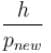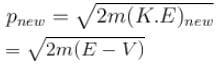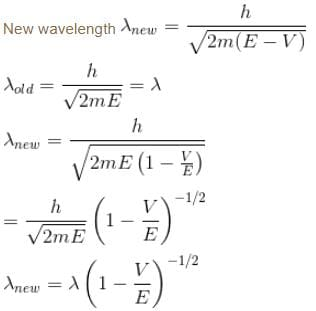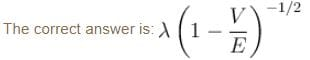Origin Of Quantum Mechanics MCQ Level - 2 - Question 8

In an experimental observation of the photoelectric effect, the stopping potential was plotted against the frequency of the incident light. Which of the following is the slope of the line?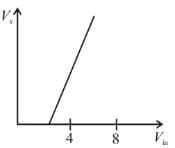Here h is the planck's constant and φ is the work function of the metal

Detailed Solution for Origin Of Quantum Mechanics MCQ Level - 2 - Question 8

If Vs is the stopping potential then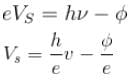Compare it with y = mx + c (equation of a straight time)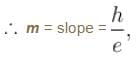c = intercept on y-axis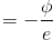Origin Of Quantum Mechanics MCQ Level - 2 - Question 9

The energy from electromagnetic waves in equilibrium in a cavity is used to melt ice. If the temperature of the cavity is raised from T to 2T (in kelvin), then the mass of ice that can be melted increases by a factor of

Detailed Solution for Origin Of Quantum Mechanics MCQ Level - 2 - Question 9

Energy radiated from a black body is proportional to the 4th power of its absolute temp is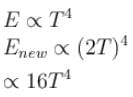Enew = 16E

Heat required to melt ice of mass m is proportional to the mass.

So, now the mass of ice that can be melted will 16 times the previous mass of ice melted.

Origin Of Quantum Mechanics MCQ Level - 2 - Question 10

If we replaced the electron in a hydrogen atom with muon, (mass of a neon is 207 times the mass of an electron) then what will be the energy levels En of this new hydrogen atom in terms of the binding energy E0 (of the ordinary hydrogen), mass of the proton mp and the principal quantum number, n? (mµ = 207 me)

Detailed Solution for Origin Of Quantum Mechanics MCQ Level - 2 - Question 10

In case the electron is replaced by the muon, we will have to consider the reduced mass of the proton- muon system.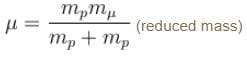The Rydberg constant is changed by a factor of.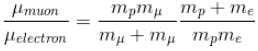The Rydbergs constant is proportional to the reduced mass of the system in consideration.

R1 = Rydbergs constant for e and p system is proportional to reduced mass of e and p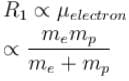R2 = Rydbergs constant for µ and p system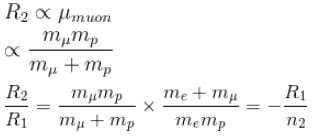∴  New energy levels are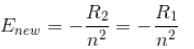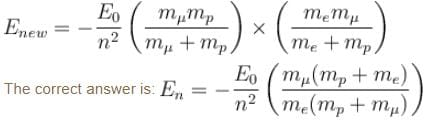## Topic wise Tests for IIT JAM Physics

217 tests
Information about Origin Of Quantum Mechanics MCQ Level - 2 Page
In this test you can find the Exam questions for Origin Of Quantum Mechanics MCQ Level - 2 solved & explained in the simplest way possible. Besides giving Questions and answers for Origin Of Quantum Mechanics MCQ Level - 2, EduRev gives you an ample number of Online tests for practice

## Topic wise Tests for IIT JAM Physics

217 tests(Scan QR code)